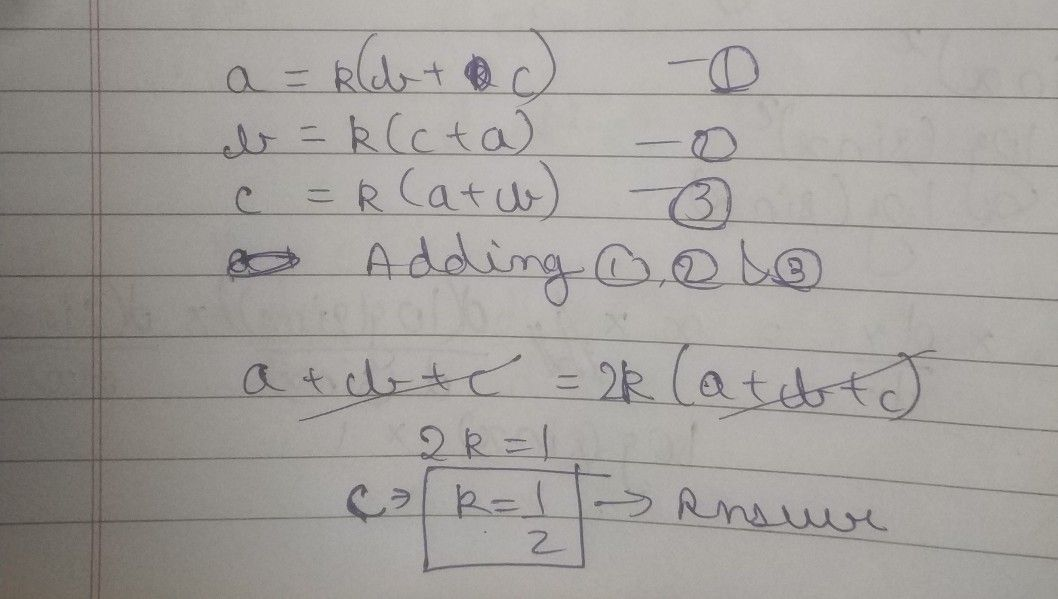Symbol
ProblemQuestion $26$ Not answered Marked out of $1.00$ $0$ $11$ $b\right)\left(c+a$ If $a,b,c>0$ and $1\left($ \frac{ $a\right)\left(b+c\right)=1+$ $=1fraC\left($ $c\right)\left(a+b\right)1\right)$ then each fraction is equal to $b=$ Select $One$ a. $114$ $○$ b. $113$ $○$ c. $112$ $O$ d. 1 $O$
Other
SolutionQanda teacher - utkarsh027best of luck☺️ please evaluate and like my profile